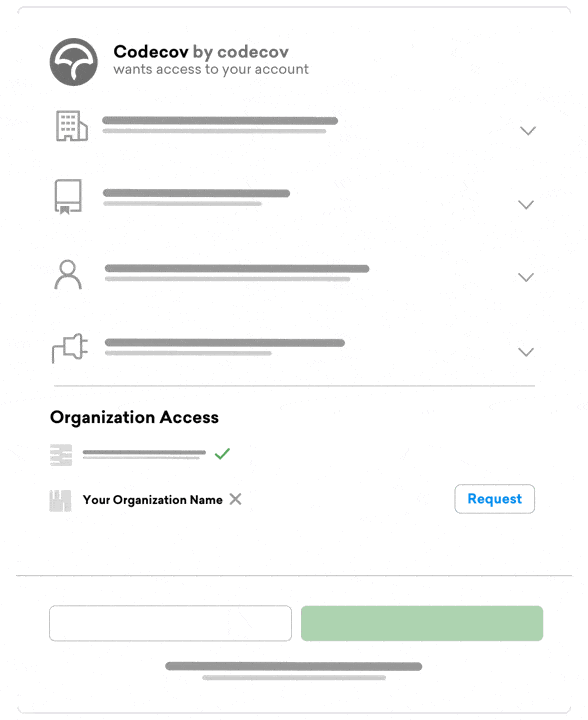# Line or Branch Coverage: Which Type of Coverage is Right for You?

October 12, 2022 Tom HuOne of the best ways to track testing on your team is with code coverage. But, there are many coverage types: line, branch, and function to name a few. Although most modern coverage tools output both line and branch coverage, it can be confusing to determine which one to use.

• what line and branch coverage are,
• how to fully cover branches,
• and which type of coverage to use (trick question, it’s both!)

## What is line coverage?

Line coverage is a basic version of test coverage. When a test suite is run, code coverage records which lines of code were hit. Line coverage, thus, is the total number of lines run divided by the number of lines in the codebase.

Take, for example, this simple calculator in `python`.

``````def add(a, b):
return a + b
def divide(a, b):
if b == 0:
return "undefined"
return a/b
``````

We have two functions, `add` and `divide`. Both take in two numbers `a` and `b`. But in the `divide` function, notice that we check to see if `b` is zero. This makes sense as we don’t want to divide by `0`.

Let’s mock out two tests, one for each function

``````def test_add():

def test_divide():
assert divide(1, 2) == 0.5
``````

If we run the tests above, we would expect the following green lines to be covered

``````def add(a, b):
return a + b
def divide(a, b):
if b == 0:
return "undefined"
return a/b
``````

Notice that the `if` statement is run, but the return below is not run. This is due to the line not being run.

In our scenario, the coverage percentage would be `83.3%` since 5 lines are run out of the 6 total.

## What is branch coverage?

Branches typically on `if` statements, when there are 2 paths to take from an evaluation. Branch coverage, thus, measures the number of branches taken over the total number of branches. Let’s revisit our example from above.

In our code, we only have one `if` statement that has two branches: `true` and `false`. Either

``````b == 0
``````

or

``````b != 0
``````

Our tests only account for the second case, so we have 50% branch coverage.

What would happen if we wanted to increase our branch coverage to 100%? We would need to run the `b == 0` case. Let’s see what our tests would look like.

``````def test_add():

def test_divide():
assert divide(1, 2) == 0.5
assert divide(1, 0) == "undefined"
``````

Now, we will be running by branches and our branch coverage would be 100%. Consequently, our line coverage would also be 100%.

## Covering branches

Our example above looks at a simple `if` statement. But not all of them have only 2 branches. For a statement like `A && (B || C)`, coverage calculation tests each possible combination of results.

 A B C Result True True True True True True False True True False True True True False False False False True True False False True False False False False True False False False False False

However, many tools will do lazy calculations as covering all cases can be cumbersome and unnecessary. In these cases, it will overlook ambiguous values. For example, our previous example would shrink down to

 A B C Result True True ? True True False True True True False False False False ? ? False

In this case, if 4 cases are written matching the above logic statements, we would see 100% branch coverage. This helps developers from having to explicitly write out all 8 paths. In this way, branch coverage can be a powerful way to account for edge cases.

## Which type of coverage should you use?

As alluded to at the start, this is a trick question. If you want to get the most out of your code coverage, you should be using both line and branch coverage. Getting line coverage is important to track that all lines are being run. But tracking branch coverage helps to make sure that you aren’t missing edge cases.

Codecov will automatically merge both types of coverage if given the information. But it’s important to note that semi-covered branches are marked as `partials` and partials are not considered hits when calculating coverage.

If you are just starting with code coverage, it might be too challenging to invest in branch coverage immediately. But if you are comfortable with your line coverage, dive into branch coverage to really hone your testing practices.

##### Before we redirect you to GitHub...